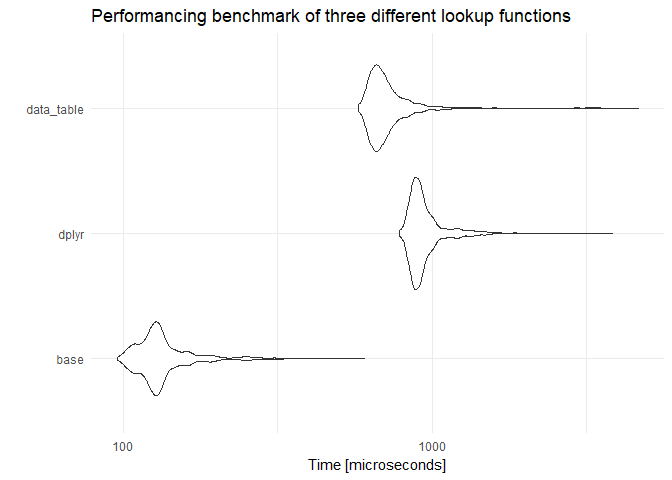# An R vlookup? Not so silly idea

It started out as a joke, but Jenny Bryan recently posted a vlookup implementation in R. Here is the original post as seen on twitter:

The argument for the creation of this kind of lookup implementation in R, was to help facilitate a join that wasn’t covered by the standard joins. This tweet started out as a joke function that would surely never see the daylight of production code, but there was one thing that stood out about the small little function - it was written in base R and made use of R’s list data objects. What makes this intriguing you ask? Well, combining lists and base usually has a nice consequence - speed.

So that is what I set out to test: How much more efficient would this vlookup be when compared to a dplyr or data.table implementation? We start off by recreating the vlookup function and use the tweet’s example to show its use case:

library(tidyverse)

vlookup <- function(this, df, key, value) {
m <- match(this, df[[key]])
df[[value]][m]
}

vlookup_base <- function(){
c("Luke Skywalker", "Jabba Desilijic Tiure", "Yoda") %>%
vlookup(starwars, "name", "mass") %>%
sum
}
vlookup_base()

##  1452


Next we build the same query, but using dplyr notation to give us the same results (a quick check shows us the sum results are the same):

vlookup_dplyr <- function(){
starwars %>%
filter(name %in% c("Luke Skywalker", "Jabba Desilijic Tiure", "Yoda")) %>%
pull(mass) %>%
sum
}
vlookup_dplyr()

##  1452


Lastly we build the query using data.table. This implementation comes from the comment section of the tweet. We first have to coerce the starwars data into a data.table object for this to work. We also lose the %>% workflow which can be quite a pain. In this case it doesn’t matter, because we all about speed!

library(data.table)
vlookup_dt <- function(){
name_vec <- c("Luke Skywalker", "Jabba Desilijic Tiure", "Yoda")
df <- data.table(dplyr::starwars)
df[.(name_vec), on = 'name', sum(mass)]
}


To test the speed to all of these function, we will use the microbenchmark library. Its one of my favourite libraries in R,thanks to its ease of use and quick API to comparing functions.

library(microbenchmark)
microbenchmark(
base = vlookup_base(),
dplyr = vlookup_dplyr(),
data_table = vlookup_dt(),
times = 1000L
)

## Unit: microseconds
##        expr      min        lq      mean   median       uq       max neval
##        base   93.539  116.1030  143.8219  129.642  147.692  4797.951  1000
##       dplyr  780.309  889.0265 1017.7738  943.795 1035.898  8516.107  1000
##  data_table 1030.564 1166.3595 1454.0140 1252.103 1441.641 50518.175  1000
##  cld
##  a
##   b
##    c


Here we see the enormous speed gain we got from using vlookup_base in comparison with the other two frameworks. In all fairness, I feel that I might be handicapping data.table a bit, just to the coercion of the starwars dataset each time. So lets see what happens when I do the coercion outside the function.

df <- data.table(dplyr::starwars)
vlookup_dt <- function(){
name_vec <- c("Luke Skywalker", "Jabba Desilijic Tiure", "Yoda")
df[.(name_vec), on = 'name', sum(mass)]
}
res_mic <- microbenchmark(
base = vlookup_base(),
dplyr = vlookup_dplyr(),
data_table = vlookup_dt(),
times = 1000L
)
res_mic

## Unit: microseconds
##        expr     min      lq     mean  median       uq      max neval cld
##        base  95.590 118.975 137.5330 128.410 140.1030  603.077  1000 a
##       dplyr 784.410 873.026 962.0909 911.180 972.7185 3840.002  1000   c
##  data_table 579.693 648.206 740.3640 685.129 746.0520 4667.900  1000  b


We definitely gained some speed by not having to coerce the data.frame over and over, but, the overall speed comparison is still nothing like the old fashion vlookup_base.